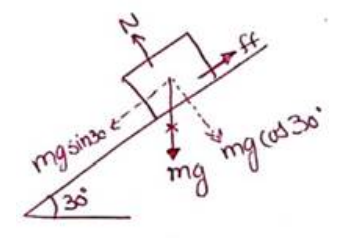# Solve the following :

Question:

A block slides down an inclined surface of inclination $30^{\circ}$ with the horizontal. Starting from rest it covers $8 \mathrm{~m}$ in the first two seconds. Fins the coefficient of kinetic friction between the two.

Solution:$N=m g \cos 30^{\circ}$

$F_{N}=m a$

$m g \sin 30-f f=m a$

$m g \sin 30-\mu_{k} N=m a$

$m g \sin 30-\mu_{k} m g \cos 30=m a$

$g \sin 30-\mu_{k} g \cos 30=a$

Now,

$u=0 ; s=8 m ; t=2 s$

$s=u t+\frac{1}{2} a t^{2}$

$8=0+\frac{1}{2}(a)(2)^{2}$

$a=4 \frac{m}{s^{2}}$

From (ii) and (iii)

$4=g \sin 30^{\circ}-\mu_{k} g \cos 30^{\circ}$

$\mu_{k}=0.11$